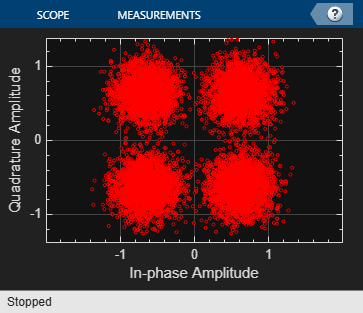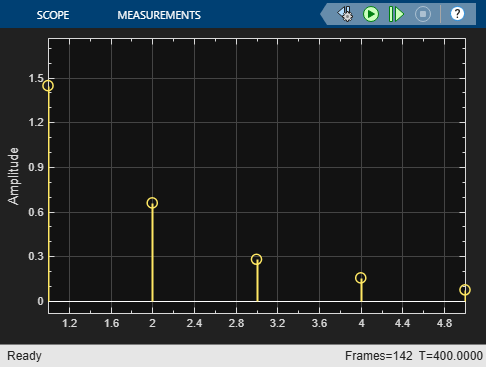# Linearly Equalize QPSK-Modulated Signal in Simulink

Apply linear equalization using the least mean squares (LMS) algorithm to recover QPSK symbols passed through an AWGN channel.The `slex_lineq_qpsk_signal` model generates an M=4 sequence using the `Random Integer Generator` block. The sequence is modulated with the `M-PSK Modulator Baseband` block, filtered with the `Discrete FIR Filter` block, and then impaired with the `AWGN` block. The `Linear Equalizer` block equalizes the data sequence, the data is demodulated with the `M-PSK Demodulator Baseband` block, and the bit error rate is computed. The signal path out of the modulator is split to a `Selector` block, which provides the first 1000 symbols of the modulated signal to the equalizer as an initial training sequence.

No delay is introduced between the transmitted and received signal because the maximum tap value is the first tap of the discrete FIR filter and the equalizer reference tap is `1`. The equalizer converges after around 1000 symbols so this value is used for the computation delay of the `Error Rate Calculation` block.

The computed error rate is displayed and plots show the equalized constellation, equalized tap weights, and signal error magnitude.

```Computed error rate = 0.0024444 ```